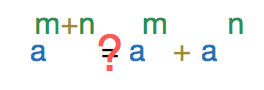# Mistakes Give Rise to Problems

Algebra Level 3We know $a^{m+n}=a^m \times a^n$ But there are some students who claim that $a^{m+n}=a^m+a^n$ which is obviously incorrect. However, it is true for some triplets of integers, $(a,m,n)$. If $a\in[0,10]$ and $m,n\in[1,10]$, then find the number of possible triplets for which $a^{m+n}=a^m+a^n$.

×

Problem Loading...

Note Loading...

Set Loading...